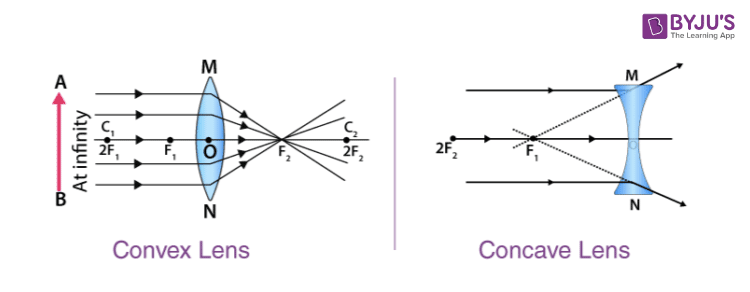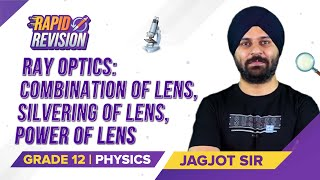Win up to 100% scholarship on Aakash BYJU'S JEE/NEET courses with ABNAT Win up to 100% scholarship on Aakash BYJU'S JEE/NEET courses with ABNAT

# Lens

Lenses are basically magnifying glasses with curved sides. A lens is a piece of transparent glass which concentrates or disperses light rays when passes through them by refraction. Due to the magnifying property, lenses are used in telescopes and other magnifying devices. They are employed in cameras for gathering light rays.

In cameras, not one but a group of lenses are used for the gathering of light. Magnification of a lens is the relation between the size of the image formed and the size of the object. Lenses can also be used in groups in order to avoid the blurriness or distortion caused to the image formed by the lens.

## Related Terms

Pole

The centre of the spherical refracting surface of the lens is called the pole. It is the point where the principal axis meets the surface of the lens.

Optical Centre

The point on the principal axis at the centre of the lens is called the optical centre.

Centre of Curvature

A lens has two spherical surfaces; these two spherical surfaces form a part of a sphere. The centre of these spheres is known as the centre of curvature.

Principal Axis

The principal axis is an imaginary line passing through the centres of curvature and the pole.

Aperture

The area of the lens suitable for refraction is called aperture. The aperture of the lens is the effective diameter of its light-transmitting area.

Focus

Focus is the point onto which collimated light parallel to the axis is focused.

Focal Length

The focal length is the distance between the optical centre and the focal point or focus of the lens

Power

The power of the lens is the reciprocal of its focal length. The SI unit of power is dioptre.

## Types of Lenses

Today, there are different types of lenses available. Generally, they are categorised either as a simple lens or a compound lens.

A simple lens is a single piece of magnifying material, while a compound lens consists of a number of simple lenses arranged along a common axis. Optical aberrations are sometimes found in simple lenses, while this property is eliminated in a compound lens. Another advantage of the compound lens is that the magnification of the lens can be adjusted as per the user’s requirements.

### Non-spherical Lenses

Aspheric Lens

An aspheric lens is often known as a non-spherical lens. An aspheric lens is a lens whose surface is not part of a sphere or a cylinder. The complex surface of an aspheric lens reduces or eliminates optical aberrations as compared to a simple lens. A single aspheric lens can replace a combination of simple lenses resulting in a system with a much-reduced size.

Cylindrical Lens

Lenses that have a curvature along only one axis are classified as cylindrical lenses. Their main purpose is to convert laser diode elliptical light into a round beam or to focus light into a line. Motion picture anamorphic lenses are an example of such lenses.

Fresnel Lens

A Fresnel lens is a lens whose optical surface is divided into narrow rings. This allows the lens to be much thinner and lighter than conventional lenses.

Other Lenses

Lenticular lenses are a group of microlenses that are used in lenticular printing. These lenses produce images that have an illusion of depth.

A bifocal lens has two or more graduated focal lengths.

A gradient index lens is a lens with flat optical surfaces, while an axicon lens features a conical optical surface.

## Concave and Convex Lens

According to the shape and purpose of the lens, they are classified into two types:

• Concave lens
• Convex lens

A ray diagram of a concave lens and the convex lens is shown below:### Concave Lens

A concave lens is a type of lens with at least one side curved inwards. A concave lens with both sides curved inward is known as a biconcave lens. Concave lenses are diverging lenses, that is, they spread out light rays that have been refracted through them. They have the ability to diverge a parallel beam of light. For a concave lens, the edges are wider than the centre, or the centre is thinner than the edges. Concave lenses are used in spectacles in order to overcome myopia or short-sightedness.

A concave lens produces a smaller image for the viewer. The focal point of a concave lens is the point from which the light rays parallel to the axis seem to diverge after passing through the lens. The distance from the optical centre of the lens to the focal point is called the focal length of the lens.

The image formed in a concave lens has the following characteristics:

• Located on the object side of the lens
• Virtual image
• Upright image
• Small in size (i.e., smaller than the object)
• The image formed in a concave lens is always in between the focal point and the optical centre. The location of the object doesn’t affect the characteristics of the image formed.

Read More: Image Formation in Convex Mirror

A concave lens always generates a virtual image. It can never form a real image. The image is always formed on the same side of the lens as the object, and thus can be seen in the lens only. It cannot be formed in a screen, and as the distance of the object from the optical centre increases, the size of the image decreases.

### Convex Lens

A convex lens is a lens with an outward curve. Unlike the concave lens, the thickness at the centre of a convex lens is more than the thickness at the edges of the lens. Convex lenses are converging lenses. They have the ability to converge a parallel beam of light into a point. This point is called the focal point of the convex lens, and the distance from the optical centre to the focal point is called the focal length. The focal point is on the opposite side of the lens from which the light rays originate.

A convex lens with one side flat is called a plano-convex lens. The lens found in the human eye is a prime example of a convex lens. Another common example of a convex lens is the magnifying glass that is used to correct hypermetropia or long-sightedness. Convex lenses are used in cameras as they focus the light and produce clear images. Convex lenses are also used in compound lenses which are employed in magnifying devices such as microscopes and telescopes.

Read More: Image Formation in Concave Mirror

## Magnification

When a linear object is placed perpendicular to the principal axis of the lens, a linear image is formed perpendicular to the principal axis due to the refraction of the lens. The position, size and nature of the image formed depend on the position of the object with respect to the lens.

The ratio of the linear size of the image to the linear size of the object is called the magnification of the lens.

m = linear size of the image/linear size of the object

## Optical Aberration

Optical aberration is a property of the lens that causes distortion or blurriness during image formation. When it occurs, the light is dispersed or spread out rather than being focused on a certain fixed point. Optical aberration is an undesirable property of lenses and can be eliminated by using a combination of lenses rather than using a single piece of lens.

There are several types of aberrations, such as spherical aberration, chromatic aberration and coma aberration, that can all affect image formation and quality.

### Spherical Aberration

One of the primary reasons for the occurrence of spherical aberration is that the spherical surfaces of the lens are not the ideal shape, and as a result, the beams are parallel to but distant from the lens axis. This causes the blurring of the image. Spherical aberration can be corrected using a normal lens having the right surface curvatures for a particular application.

### Coma Aberration

When an object is imaged off the optical axis of the lens, coma aberration takes place. Coma aberration can be eliminated by taking the curvature of the two lens surfaces that matches the application. Best form lenses can be used for it.

### Chromatic Aberration

Chromatic aberration occurs due to dispersion. When it occurs, a lens fails to focus all colours on the same point. Chromatic aberration can be observed as colourful fringes around an image. It can be fixed using an achromatic doublet (or achromat).

There are other types of aberrations, and some of them include field curvature, astigmatism, and barrel and pincushion distortion.

## Uses of Lens

• Used in a magnifying glass.
• Prosthetics for the correction of visual impairments.
• Attenuate light.
• Used in imaging systems.

## Related Videos## Frequently Asked Questions on Lens

Q1

### Define the power of a lens.

The power of a lens is a measure of its ability to converge or diverge the incident beam of light. It is given by the reciprocal of focal length.

Q2

### Explain the term focal length.

The distance between the optic centre and the principal focal is called the focal length.

Q3

### What is a convex lens?

A lens that is thinner in the centre than at the edges and converges a beam of light is called a convex lens.

Q4

### What is a concave lens?

A concave lens is thicker at the centre than at the edges, and it diverges the beam of light.